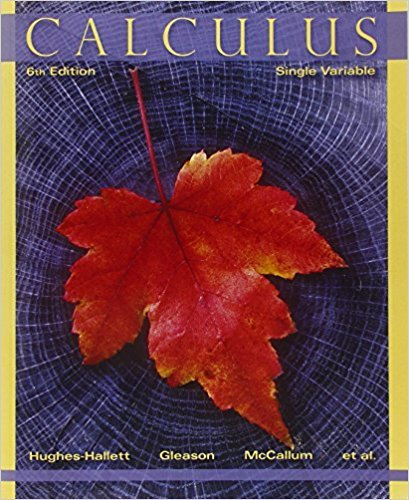×
×

# Solutions for Chapter 9.2: GEOMETRIC SERIES## Full solutions for Calculus: Single Variable | 6th Edition

ISBN: 9780470888643Solutions for Chapter 9.2: GEOMETRIC SERIES

Solutions for Chapter 9.2
4 5 0 323 Reviews
27
0
##### ISBN: 9780470888643

This expansive textbook survival guide covers the following chapters and their solutions. Chapter 9.2: GEOMETRIC SERIES includes 54 full step-by-step solutions. This textbook survival guide was created for the textbook: Calculus: Single Variable , edition: 6. Calculus: Single Variable was written by and is associated to the ISBN: 9780470888643. Since 54 problems in chapter 9.2: GEOMETRIC SERIES have been answered, more than 35473 students have viewed full step-by-step solutions from this chapter.

Key Calculus Terms and definitions covered in this textbook
• Additive inverse of a complex number

The opposite of a + bi, or -a - bi

• Cofunction identity

An identity that relates the sine, secant, or tangent to the cosine, cosecant, or cotangent, respectively

• Continuous function

A function that is continuous on its entire domain

• Deductive reasoning

The process of utilizing general information to prove a specific hypothesis

• Difference identity

An identity involving a trigonometric function of u - v

• Double-angle identity

An identity involving a trigonometric function of 2u

• Inverse tangent function

The function y = tan-1 x

• Lower bound test for real zeros

A test for finding a lower bound for the real zeros of a polynomial

• Negative angle

Angle generated by clockwise rotation.

• Outcomes

The various possible results of an experiment.

• Polar coordinates

The numbers (r, ?) that determine a point’s location in a polar coordinate system. The number r is the directed distance and ? is the directed angle

• Product of functions

(ƒg)(x) = ƒ(x)g(x)

• Real zeros

Zeros of a function that are real numbers.

• Relation

A set of ordered pairs of real numbers.

• Root of a number

See Principal nth root.

• Secant line of ƒ

A line joining two points of the graph of ƒ.

• Solve algebraically

Use an algebraic method, including paper and pencil manipulation and obvious mental work, with no calculator or grapher use. When appropriate, the final exact solution may be approximated by a calculator

• Symmetric matrix

A matrix A = [aij] with the property aij = aji for all i and j

• Vertical component

See Component form of a vector.

• Window dimensions

The restrictions on x and y that specify a viewing window. See Viewing window.

×